The ADDRESS Google Sheets function returns a cell’s address in the form of a text. It is noteworthy that the resulting address is a string and not the cell reference.

## Syntax

• row – this value indicates the row number where the cell, that we need the address for, is located.
• column – just like the row parameter, this specifies the column number where the cell, that we need the address for, is located.
• absolute_relative_mode – this optional parameter accepts four different values 1, 2, 3 and 4. Each of these four values represents one of the four different modes of cell references. We explain this in the table below. If we do not specify anything against this parameter, the ADDRESS function considers 1 as the default input.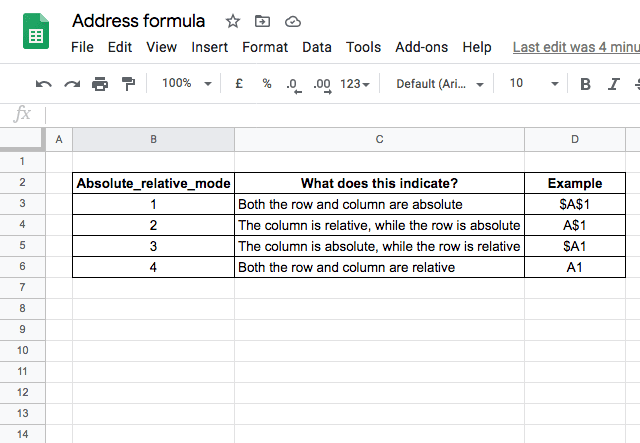• use_a1_notation – there are two kinds of representations for a cell address. One is the A1 format, which gives the column as a letter and the row as a number (e.g. B4, D11, etc.). And the other way of representing the same cell is R1C1 format (which gives the row number and column number, e.g. R1C5, R3C2, etc.). TRUE is the value by default, and you get A1 notation in return. Otherwise, if you need R1C1 notation, you need to specify FALSE.
• sheet – is another optional parameter, that is the name of the sheet where the cell address points to. Accordingly, the function includes this name in the fully qualified address that it returns.

## How to use the ADDRESS function

As is the case with all functions and formulas, the best way to understand ADDRESS is to see it in use.

Below are a few combinations of the function.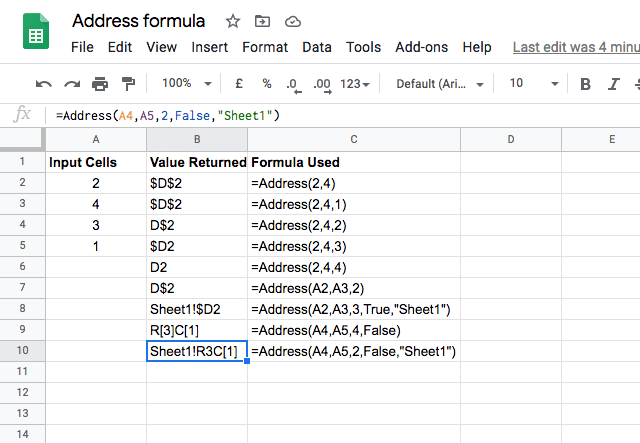In the snapshot above, notice that the R1C1 style notation has brought in square brackets [ and ] in rows 9 and 10. Please note that a value within the square bracket indicates either a relative row or column. So, for instance, if the result of the formula is R3C, that translates to this: 3rd row is absolute while the 1st column is relative.

So far, so good. Now, is there a way that we can use the output from the ADDRESS Google Sheets function as a cell reference, given that it returns only a text? Sure, there is! Use this output as an input to the INDIRECT function, and voila, we have the cell reference with us!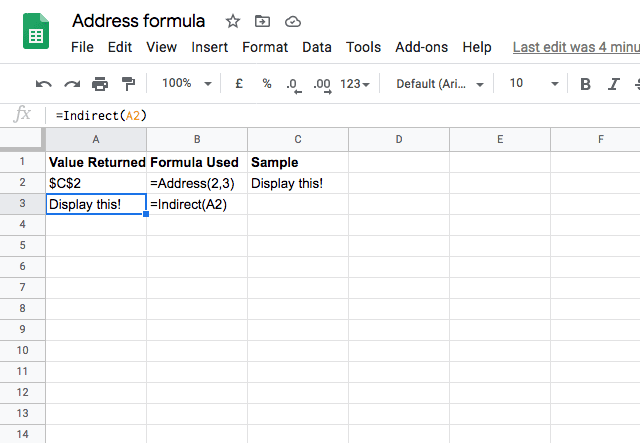### Translate column numbers to column letters

The ADDRESS function can also be used to translate column numbers into column letters. Say you have a list of columns in numerical format, and want to translate these into column letters – this can be achieved by combining the ADDRESS and SUBSTITUTE functions.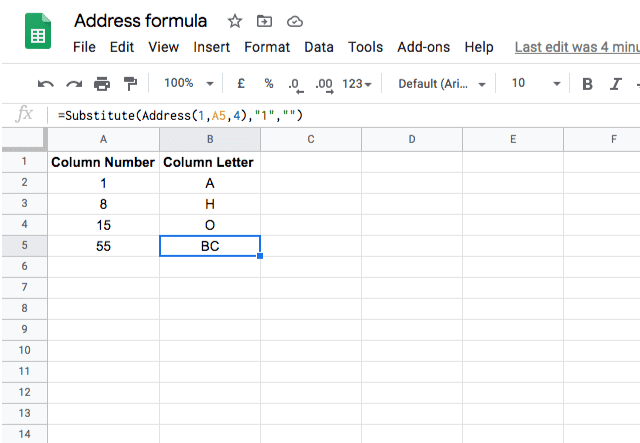As you can see above, the ADDRESS function provides the cell reference, and the SUBSTITUTE function substitutes the row number (1) for an empty text string. What remains is the column letter! This is achieved with the formula =Substitute(Address(1,A5,4),”1″,””).

### Find the address of a particular value

Another use of the address is when you have a long list of data, and you want to know the address of a particular value. In the example below, I use the MATCH function alongside the ADDRESS function to find the address of a particular value. While the dataset is small in the example, you can imagine that it could be helpful in a very large dataset when you are searching for a single data point.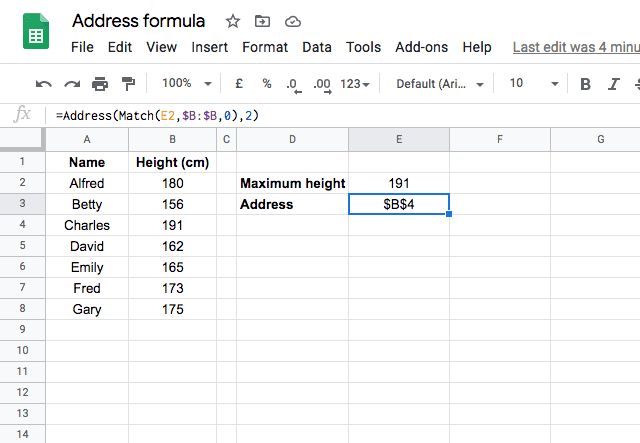The MATCH function identifies the location of the cell that contains the largest height, and the ADDRESS function returns the address of this cell. This is done by typing =Address(Match(E2,\$B:\$B,0),2).

### Address of the minimum value

I’ll end this topic with one last example. Here’s an interesting use case for finding out the address of the cell that has minimum sales figures.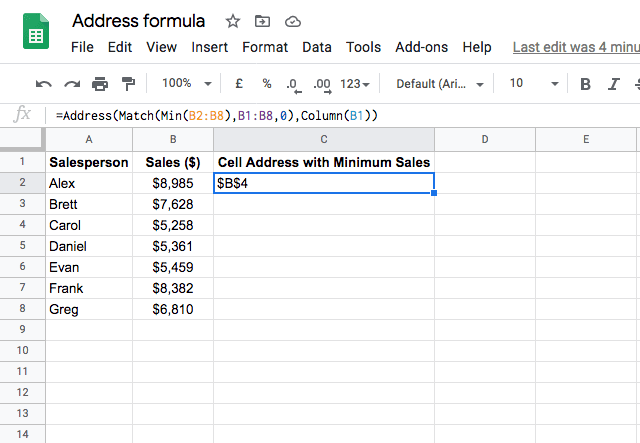That’s how to get started with Google Sheets ADDRESS function. Looking for more spreadsheet tutorials and tips? Check out our other posts below!

Editor’s note: This is a revised version of a previous post that has been updated for accuracy and comprehensiveness.